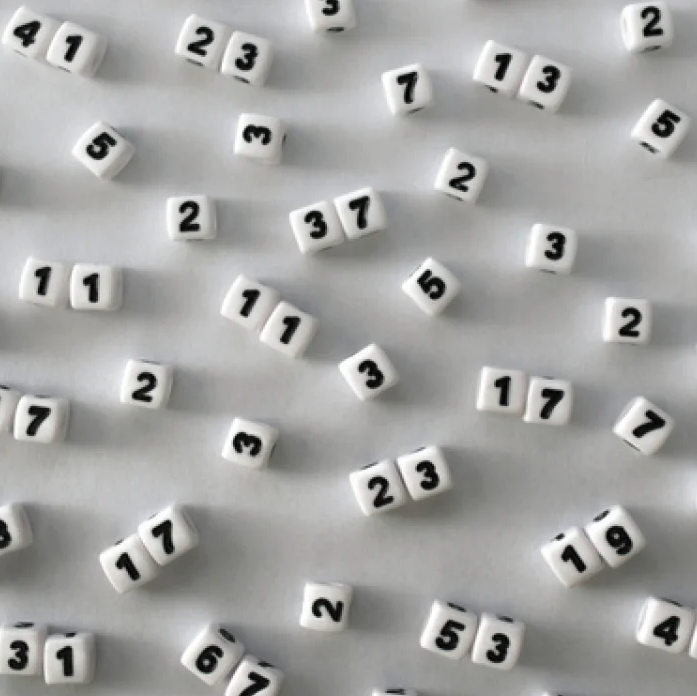# Random Chowlaconjecture

Number theory

The distribution of partial sums of a Steinhaus random multiplicative function, of polynomials in a given form, converges to the standard complex Gaussian.

## On the random Chowla conjecture

We show that for a Steinhaus random multiplicative function $f:\mathbb{N}\rightarrow\mathbb{D}$ and any polynomial $P(x)\in\mathbb{Z}[x]$ of $\deg P \geq 2$ which is not of the form $w(x+c)^d$ for some $w \in \mathbb{Z}, c \in \mathbb{Q}$, we have $1/\sqrt{x} \cdot \sum_{n\leq x} f(P(n)) \xrightarrow{d} \mathcal{C} \mathcal{N}(0,1)$, where $\mathcal{C}\mathcal{N}(0,1)$ is the standard complex Gaussian distribution with mean 0 and variance 1. This confirms a conjecture of Najnudel in a strong form. We further show that there almost surely exist arbitrary large values of $x \geq 1$, such that $|\sum_{n\leq x} f(P(n))| \gg_{\deg P} \sqrt{x}(\log \log x)^{1/2},$ for any polynomial $P(x)\in\mathbb{Z}[x]$ with $\deg P \geq 2$, which is not a product of linear factors (over $\mathbb{Q}$). This matches the bound predicted by the law of the iterated logarithm. Both of these results are in contrast with the well-known case of linear phase $P(n) = n,$ where the partial sums are known to behave in a non-Gaussian fashion and the corresponding sharp fluctuations are speculated to be $O(\sqrt{x}(\log\log x)^{1/4 + \varepsilon})$ for any $\varepsilon > 0$.